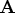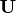Previous Page | Next Page

 Language Reference

## ROOT Function

performs the Cholesky decomposition of a matrix

ROOT( matrix)

where matrix is a symmetric positive-definite matrix.

The ROOT function performs the Cholesky decomposition of a matrix (for example,) such thatwhereis upper triangular. The matrixmust be symmetric and positive definite.

For example, consider the following statements:

```
xpx={25 0 5, 0 4 6, 5 6 59};
U=root(xpx);
```
These statements produce the following result:
```
U

5         0         1
0         2         3
0         0         7
```

If you need to solve a linear system and you already have a Cholesky decomposition of your matrix, then use the TRISOLV function as illustrated in the following code.

```
b = {5, 2, 53};
/* want to solve xpx * t = b.
First solve U` z = b,
then solve U t = z */
z = trisolv(2,U,b);
t = trisolv(1,U,z);
```
The solution is as follows:
```
T

0
-1
1
```

The ROOT function performs most of its computations in the memory allocated for returning the Cholesky decomposition.Previous Page | Next Page | Top of Page